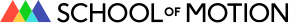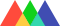#School of Motion## What is Premultiplication?

• By Joey Korenman
• Share

Howdy folks!
I got the awesome opportunity to partner with The Foundry on a couple of videos to help explain topics that might trip up After Effects artists who are starting to use Nuke. Watch these 2 short videos first, and then keep reading if you’re a geek-type and want to really see how the sausage gets made.

## Managing Premultiplication

If that wasn’t enough, check out this guide that goes a bit deeper into the math (that’s right… MATH) behind compositing.  I tried to make it interesting, but let’s be honest… it’s premultiplication.  It’s just not sexy.
Let’s start by talking about how compositing programs actually… ya know… composite.  Let’s say you have two images, A and B.
B will be our background image, and A will be our foreground.  As it turns out, A has an alpha channel… we’ll call this alpha channel “a.”
If you create a Merge node in Nuke and hover your mouse over the Operation option, you’ll see a CRAZY looking sheet pop up that looks a lot like an Algebra test.  This is actually a list of the mathematical formulas that each composite mode is using inside of a Merge node.
Let’s look at the formula for a basic “Over” operation… this is just layering one image over another.
I know… WTF!?!?  Hang tight, it will all make sense.  What that formula means, is that in order to figure out what the new COMBINED image looks like, we have to do some math using the two SOURCE images.  Not fancy math either… plain old addition and multiplication.  Here is what that formula looks like image-wise:
Let’s start with the (1-a) part of the equation.  What is 1 minus an image?  It makes no sense!!!  Actually, all we are doing is looking at the COLOR VALUE of each pixel in the alpha channel (White = 1, Black = 0, Grey = .5) and subtracting that number from 1 to get the new value.  When you do this, you invert the alpha channel and get…
Ok, so now our math formula looks like this:
Now we can multiply B by the inverted alpha channel.  How do we do that?  Well, for the purposes of this example I chose a blue that has RGB values of R=.2, G=.2, B=1.
(side note: Nuke works in 32-bit mode, so color values go from 0-1, not 0-255 like you may be used to seeing in After Effects’ 8-bit default mode.  The principal is the same in that app as well)
We are going to multiply every one of B’s pixel’s COLOR VALUE times the values of the pixels in the inverted alpha.  So, again, a blue pixel times a black pixel (remember, black=0) will equal a black pixel (R=0, G=0, B=0).  A blue pixel times a white pixel (white = 1) equals an unchanged blue pixel.
It’s slightly more interesting when we look at the grayish pixels along the edges of the alpha, the ones that are not black or white, but are somewhere in the middle because of antialiasing.
One of those pixels might have a value of .5, so a blue pixel times a .5 pixel would equal something like this:
The new pixel has a value of R=.1, G=.1, B=.5.  It has been darkened in the multiplication process.  THIS IS IMPORTANT.  It has NOT been made transparent, it has been darkened.  The result of this multiplication looks like this:
You might say, “Well shoot!  That looks like what you’d get if you used the Multiply blend mode in Photoshop or After Effects,” and then I would say, “Damn straight.”  There is also an Add mode in those two apps… care to guess what it does?  So now, we’re left with this piece of the formula.
By now I’m sure you can figure out what it means to ADD these two images together, so let’s do it and find out what happens!  For reference, the yellow color I chose has a value of R=.9, G=.9, B=.2.  We add them together and….